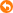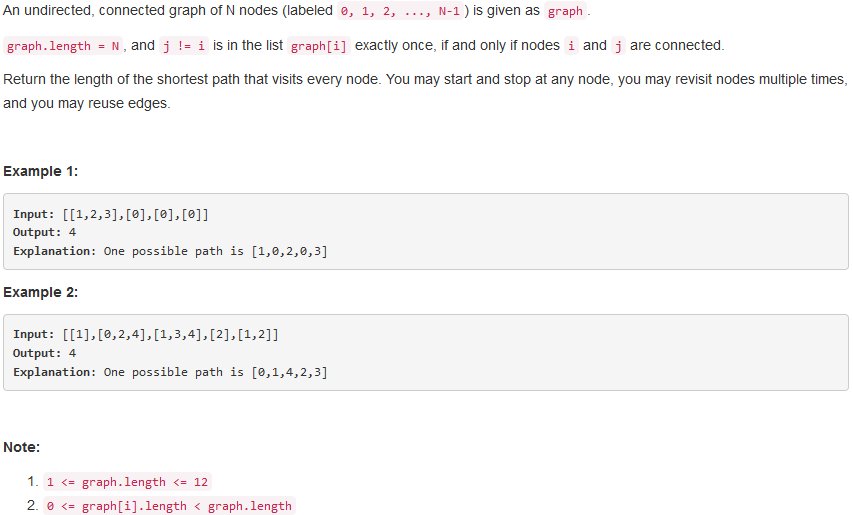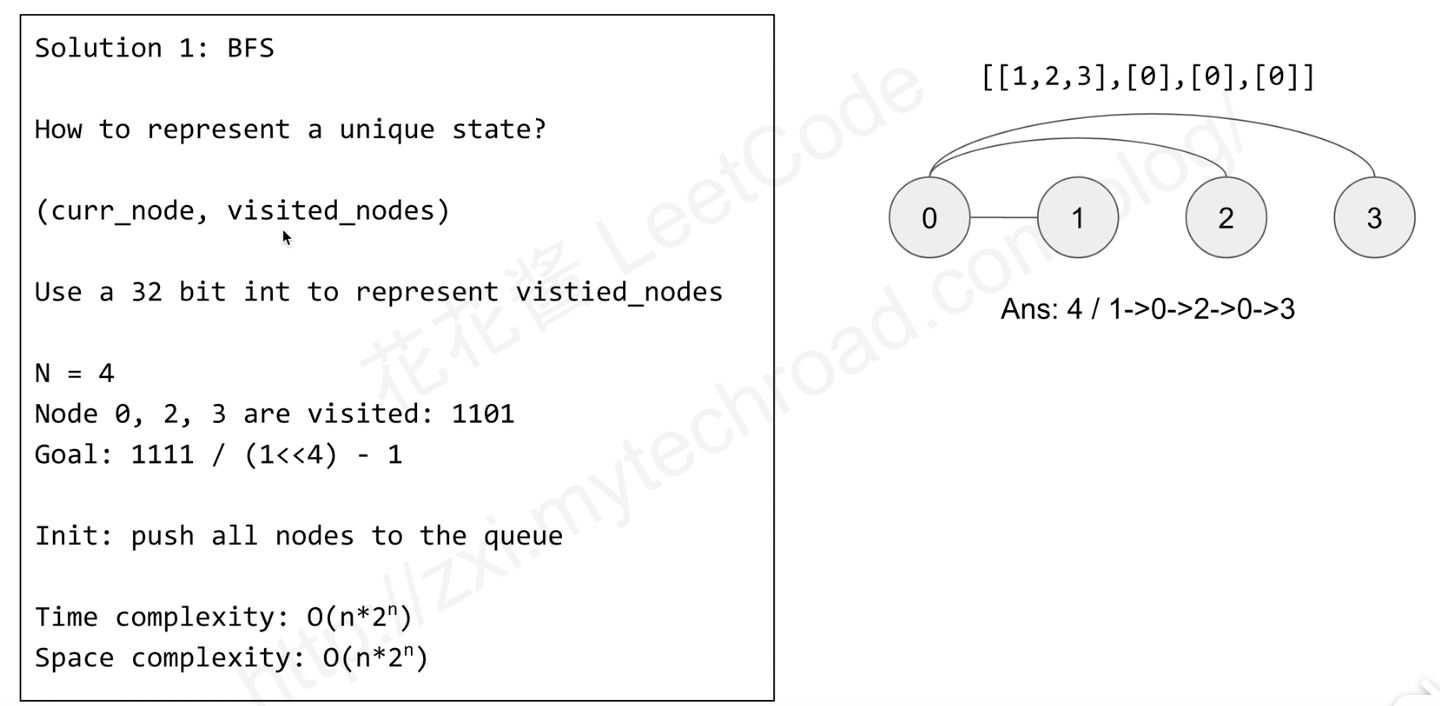• zjw123asd 发表于 2012-4-20 20:30 题目就是如上所示function r = geodistance( ci , cf , m )%GEODISTANCE: Calculates the distance in meters between two points on earth surface.%% Usage:r = geodistance( ...
zjw123asd 发表于 2012-4-20 20:30题目就是如上所示function r = geodistance( ci , cf , m )%GEODISTANCE: Calculates the distance in meters between two points on earth surface.%% Usage:  r = geodistance( coordinates1 , coordinates2 , method ) ;%%          Where coordinates1 = [lambda1,phi1] defines the%          initial position and coordinates2 = [lambda2,phi2]%          defines the final position.%          Coordinates values should be specified in decimal degrees.%          Method can be an integer between 1 and 23, default is m = 6.%         Methods 1 and 2 are based on spherical trigonometry and a%         spheroidal model for the earth, respectively.%          Methods 3 to 24 use Vincenty's formulae, based on ellipsoid%         parameters.%         Here it follows the correspondence between m and the type of%         ellipsoid:%%         m =  3 -> ANS ,        m =  4 -> GRS80,    m = 5 -> WGS72,%         m =  6 -> WGS84,       m =  7 -> NSWC-9Z2,%         m =  8 -> Clarke 1866, m =  9 -> Clarke 1880,%         m = 10 -> Airy 1830,%         m = 11 -> Bessel 1841 (Ethiopia,Indonesia,Japan,Korea),%         m = 12 -> Bessel 1841 (Namibia),%         m = 13 -> Sabah and Sarawak (Everest,Brunei,E.Malaysia),%         m = 14 -> India 1830, m = 15 -> India 1956,%         m = 16 -> W. Malaysia and Singapore 1948,%         m = 17 -> W. Malaysia 1969,%         m = 18 -> Helmert 1906, m = 19 -> Helmert 1960,%         m = 20 -> Hayford International 1924,%         m = 21 -> Hough 1960, m = 22 -> Krassovsky 1940,%         m = 23 -> Modified Fischer 1960,%         m = 24 -> South American 1969.%%          Important notes:%%         1)South latitudes are negative.%         2)East longitudes are positive.%         3)Great circle distance is the shortest distance between two points%          on a sphere. This coincides with the circumference of a circle which%          passes through both points and the centre of the sphere.%         4)Geodesic distance is the shortest distance between two points on a spheroid.%         5)Normal section distance is formed by a plane on a spheroid containing a%          point at one end of the line and the normal of the point at the other end.%          For all practical purposes, the difference between a normal section and a%          geodesic distance is insignificant.%         6)The method m=2 assumes a spheroidal model for the earth with an average%          radius of 6364.963 km. It has been derived for use within Australia.%          The formula is estimated to have an accuracy of about 200 metres over 50 km,%          but may deteriorate with longer distances.%          However, it is not symmetric when the points are exchanged.%%  Examples: A = [150 -30]; B = [150 -31]; L = [151 -80];%            [geodistance(A,B,1) geodistance(A,B,2) geodistance(A,B,3)]%            [geodistance(A,L,1) geodistance(A,L,2) geodistance(A,L,3)]%            geodistance([0 0],[2 3])%            geodistance([2 3],[0 0])%            geodistance([0 0],[2 3],1)%            geodistance([2 3],[0 0],1)%            geodistance([0 0],[2 3],2)%            geodistance([2 3],[0 0],2)%            for m = 1:24%            r(m) = geodistance([150 -30],[151 -80],m);%            end%            plot([1:m],r), box on, grid on%***************************************************************************************% Second version: 07/11/2007% Third  version: 03/08/2010%% Contact: orodrig@ualg.pt%% Any suggestions to improve the performance of this% code will be greatly appreciated.%% Reference: Geodetic Calculations Methods%            Geoscience Australia%%%***************************************************************************************r = [ ];if nargin == 2, m = 6; endlambda1 = ci(1)*pi/180;phi1 = ci(2)*pi/180;lambda2 = cf(1)*pi/180;phi2 = cf(2)*pi/180;L = lambda2 - lambda1;alla = [0 0 6378160 6378137.0 6378135 6378137.0 6378145 6378206.4 6378249.145,...6377563.396 6377397.155 6377483.865,...6377298.556 6377276.345 6377301.243 6377304.063 6377295.664 6378200 6378270 6378388  6378270 6378245,...6378155 6378160];allf = [0 0  1/298.25 1/298.257222101 1/298.26 1/298.257223563 1/298.25 1/294.9786982 1/293.465,...1/299.3249646 1/299.1528128,...1/299.1528128 1/300.8017 1/300.8017 1/300.8017 1/300.8017 1/300.8017 1/298.3 1/297 1/297 1/297,...1/298.3 1/298.3 1/298.25];if ( lambda1 == lambda2 )&( phi1 == phi2 )r = 0;elseif m == 1 % Great Circle Distance, based on spherical trigonometryr = 180*1.852*60*acos( ...sin(phi1)*sin(phi2) + cos(phi1)*cos(phi2)*cos(lambda2-lambda1) )/pi;r = 1000*abs( r );elseif m == 2 % Spheroidal model for the earthterm1 = 111.08956*( ci(2) - cf(2) + 0.000001 );term2 = cos( phi1  + ( (phi2 - phi1)/2 ) );term3 = ( cf(1) - ci(1) + 0.000001 )/( cf(2) - ci(2) + 0.000001 );r = 1000*abs( term1/cos( atan( term2*term3 ) ) );else % Apply Vincenty's formulae (as long as the points are not coincident):a = alla(m);f = allf(m);b = a*( 1 - f );axa = a^2;bxb = b^2;U1 = atan( ( 1 - f )*tan( phi1 ) );U2 = atan( ( 1 - f )*tan( phi2 ) );lambda     =  L;lambda_old = sqrt(-1); % There is no way a complex number is going to coincide with a real number!ntrials = 0; % Just in case...while ( abs( lambda - lambda_old ) > 1e-9 )ntrials = ntrials + 1;lambda_old = lambda;sin_sigma = sqrt( ( cos(U2)*sin(lambda) )^2 + ( cos(U1)*sin(U2) - sin(U1)*cos(U2)*cos(lambda) )^2 );cos_sigma = sin( U1 )*sin( U2 ) + cos( U1 )*cos( U2 )*cos( lambda );sigma = atan2( sin_sigma,cos_sigma );sin_alpha = cos( U1 )*cos( U2 )*sin( lambda )/sin_sigma;cos2_alpha = 1 - sin_alpha^2;cos_2sigmam = cos_sigma - 2*sin( U1 )*sin( U2 )/cos2_alpha;C = (f/16)*cos2_alpha*( 4 + f*( 4 - 3*cos2_alpha ) );lambda  = L + ( 1 - C )*f*sin_alpha*( sigma + C*sin_sigma*( ...cos_2sigmam + C*cos_sigma*( -1 + 2*( cos_2sigmam )^2 ) ) );%Stop the function if convergence is not achieved:if ntrials > 1000disp('Convergence failure...')returnendend% Convergence achieved? get the distance:uxu = cos2_alpha*( axa - bxb )/bxb;A = 1 + ( uxu/16384 )*( 4096 + uxu*( -768 + uxu*( 320 - 175*uxu ) ) );B = ( uxu/1024 )*( 256 + uxu*( -128 + uxu*( 74 - 47*uxu ) ) );delta_sigma = B*sin_sigma*( cos_2sigmam + ( B/4 )*(  ...cos_sigma*( -1 + 2*cos_2sigmam^2 ) - ...(B/6)*cos_2sigmam*( -3 + 4*sin_sigma^2 )*( -3 + 4*cos_2sigmam^2 ) ) );r = b*A*( sigma - delta_sigma );endend
展开全文• 图的遍历：指从图的某一顶点出发，按照某种搜索方法沿着图中的边，对所有节点访问一次且仅一次。 图的遍历主要有两种算法：广度优先遍历和深度优先遍历 这两种遍历算法，我在考研期间有复习过，所以现在写到博客上...
图的遍历：指从图的某一顶点出发，按照某种搜索方法沿着图中的边，对所有节点访问一次且仅一次。

图的遍历主要有两种算法：广度优先遍历和深度优先遍历

这两种遍历算法，我在考研期间有复习过，所以现在写到博客上（算法的实现前提是都是基于图的邻接表的存储方式存储的）

我们知道，通常图的存储方式有两种：邻接矩阵和邻接表，本篇中的存储方式则是基于邻接表的方式存储的。

存储结构：

typedef struct ArcNode{//边表结点
int adjex;//该边所指向的下一个顶点的位置
struct ArcNode *next;//指向下一条边的指针
}ArcNode;
typedef struct VNode{
int data;//存放顶点信息
ArcNode *firstarc;//指向第一个边表结点的指针
}VNode,Adjlist;
typedef struct{
Adjlist adjlist;//存放顶点表的数组
int vernum,arcnum;//存放的顶点数和边数
}Graph;

图的广度优先遍历

思想：

首先给定访问的初始顶点v，由该顶点v出发，依次访问与它相邻且未访问过的节点w1,w2,w3........wi,直至访问完。然后再依次访问与w1相邻且未访问过的节点，以此类推，直至所有的节点都访问过了。

实现代码：

#include<iostream>
#include<queue>
using namespace std;

bool visited;
//该算法是使用到了图的邻接表的存储方法实现的

typedef struct ArcNode{//边表结点
int adjex;//该边所指向的下一个顶点的位置
struct ArcNode *next;//指向下一条边的指针
}ArcNode;
typedef struct VNode{
int data;//存放顶点信息
ArcNode *firstarc;//指向第一个边表结点的指针
}VNode,Adjlist;
typedef struct{
Adjlist adjlist;//存放顶点表的数组
int vernum,arcnum;//存放的顶点数和边数
}Graph;
void visit(Graph G,int v){
cout<<"现在访问的节点是"<<(G.adjlist[v]).data<<endl;
}
//广度优先遍历
void BFS(Graph G,int v){
visit(G,v);//访问起始结点v
visited[v]=true;//置访问标记
queue<int> q;//声明定义队列q
q.push(v);//将已访问过的顶点v入队列
while(!q.empty()){//队列不为空时
v=q.front();//取队首元素
q.pop();//出队列
ArcNode *p=(G.adjlist[v]).firstarc;//p指向其第一个边表结点处
while(p){//p不为空时
if(!visited[p->adjex]){//该顶点未被访问过时
visit(G,p->adjex);
visited[v]=true;
q.push(p->adjex);
}
else p=p->next;//若该边已被访问过，则指向下一条
}
}
}
void BFS_Traverse(Graph G){
int i;
for(i=0;i<G.vernum;i++){
visited[i]=false;
}
for(int j=0;j<G.vernum;j++){
if(!visited[j]){
BFS(G,i);}
}
}

同时，基于广度优先遍历的实例也有很多，比如求单源最短路径（即求从一个顶点出发，到其它顶点的最短路径问题）

思想：

设置数组d[i]用于记录到每个顶点的距离，开始时将其初始化为无穷大，并设置起始顶点的d[v]=0,利用广度优先遍历算法，所有与v顶点相邻接的顶点i,d[i]=d[v]+1;以此类推，具体实现如下：

//单源最短路径
void BFS_Min(Graph G,int v){
int d;
for(int i=0;i<G.vernum;i++){//置数组中的每个元素的d[i](标识距离)都为无穷大
d[i]=9999;
}
visit(G,v);
visited[v]=true;
d[v]=0;//因为是计算的v到其他节点的距离，所以v到自己的距离为0
queue<int> q2;
q2.push(v);
while(!q2.empty()){
v=q2.front();
q2.pop();
ArcNode *p2=(G.adjlist[v]).firstarc;//指向第一个边表结点
if(p2){
visit(G,p2->adjex);
visited[p2->adjex]=true;
d[p2->adjex]=d[v]+1;
q2.push(p2->adjex);
}
else p2=p2->next;
}
}


深度优先遍历算法：

思想：

尽可能深的搜索，由起始顶点v出发，访问与v邻接且未被访问的任意顶点w1,再访问与w1邻接且未被访问的任意顶点w2,依次访问，直至全部都访问到。

我们采用递归的方式实现，先访问起始顶点，并置访问标记，定义指针p指向该节点的第一个边表结点

当p未被访问过时，递归调用DFS算法进行访问，当p已被访问过，则执行p=p->next,移到下一个边表结点。

实现：

//深度优先遍历
//采用递归的方法实现
void DFS(Graph G,int v){
visit(G,v);
visited[v]=true;
ArcNode *p=(G.adjlist[v]).firstarc;//定位到第一个边表结点
if(!visited[p->adjex]){//如果改变没有访问过，则递归调用dfs访问
DFS(G,p->adjex);
}
}

以上就是图的遍历的两种算法的实现

展开全文数据结构
• 经分析我们发现，只有一条路径上的边可以少走一次，这是很容易想通的，因为你走到“叶子节点”的话，倘若此时还有节点没有遍历到，呢你肯定是要拐回去的，因此我们只需要找到一条最长的路径，只走一次这条路径就好了...
链接：https://ac.nowcoder.com/acm/contest/188/C?&headNav=www
来源：牛客网

题目描述

小w不会离散数学，所以她van的图论游戏是送分的

小w有一张n个点n-1条边的无向联通图,每个点编号为1~n,每条边都有一个长度
小w现在在点x上
她想知道从点x出发经过每个点至少一次，最少需要走多少路

输入描述:

第一行两个整数 n,x,代表点数,和小w所处的位置
第二到第n行,每行三个整数 u,v,w,表示u和v之间有一条长为w的道路

输出描述:

一个数表示答案

示例1

输入

复制3 1 1 2 1 2 3 1

3 1
1 2 1
2 3 1

输出

复制2

2

备注:

1 ≤ n ≤ 50000 , 1 ≤ w ≤ 2147483647

思路：最差情况下，所走的路径长度一定是所有边的长度之和乘2，但是由于我们不需要每次都返回起点，因此我们可以少走些冤枉路，在这张图上，一定有一些点是属于“叶子节点”的，这里的“叶子节点”是指该节点只有一个相邻节点，并且不是起点。经分析我们发现，只有一条路径上的边可以少走一次，这是很容易想通的，因为你走到“叶子节点”的话，倘若此时还有节点没有遍历到，呢你肯定是要拐回去的，因此我们只需要找到一条最长的路径，只走一次这条路径就好了。

import javax.management.loading.MLetMBean;
import javax.swing.plaf.synth.SynthTextAreaUI;
import java.awt.*;
import java.io.CharConversionException;
import java.lang.reflect.Array;
import java.sql.SQLOutput;
import java.util.*;
import java.util.List;

public class Main {

static class node {
int v, w;

public node(int v, int w) {
this.v = v;
this.w = w;
}
}

private static int ans;
private static boolean[] flag;
private static List<List<node>> list;

public static void main(String[] args) {

ans = 0;
int sum = 0;
int n, x, u, v, w;
Scanner in = new Scanner(System.in);

n = in.nextInt();
x = in.nextInt();
flag = new boolean[n + 1];
list = new ArrayList<>();
for (int i = 0; i <= n; i++)
list.add(new ArrayList<>());

for (int i = 0; i < n - 1; i++) {
u = in.nextInt();
v = in.nextInt();
w = in.nextInt();
list.get(u).add(new node(v, w));
list.get(v).add(new node(u, w));
sum += w;
}

dfs(x, 0);

System.out.println(sum * 2 - ans);

}

private static void dfs(int x, int dis) {

flag[x] = true;
int size = list.get(x).size();

if (size == 1 && flag[list.get(x).get(0).v]) {
ans = Math.max(ans, dis);
return;
}

for (int i = 0; i < size; i++) {
int nxt = list.get(x).get(i).v;
if (flag[nxt]) continue;
dfs(nxt, dis + list.get(x).get(i).w);
}

}
}


展开全文• 本题要求是求遍历所有节点最短路径，由于本题中是没有要求一个节点只能访问一次的，也就是说可以访问一个节点多次，但是如果表征两次节点状态呢？可以使用（curNode， VisitedNode）来进行表征，如果两次的已经...

2018-10-06 22:04:38
问题描述：问题求解：
本题要求是求遍历所有节点的最短路径，由于本题中是没有要求一个节点只能访问一次的，也就是说可以访问一个节点多次，但是如果表征两次节点状态呢？可以使用（curNode， VisitedNode）来进行表征，如果两次的已经访问的节点相同那么就没有必要再进行访问了，最终的状态就是所有节点都访问过了。另外，由于起点对结果是有影响的，因此在最开始需要将所有的节点都压栈。

    public int shortestPathLength(int[][] graph) {
int n = graph.length;
int[][] used = new int[n][1 << n];
Queue<int[]> q = new LinkedList<>();
for (int i = 0; i < n; i++) {
used[i][1 << i] = 1;
q.add(new int[]{i, 1 << i});
}
int step = 0;
while(!q.isEmpty()) {
int size = q.size();
for (int i = 0; i < size; i++) {
int[] curNode = q.poll();
int node = curNode;
int state = curNode;
if (state == ((1 << n) - 1)) return step;
for (int k : graph[curNode]) {
int newState = state | (1 << k);
if (used[k][newState] == 1) continue;
used[k][newState] = 1;
q.add(new int[]{k, newState});
}
}
step++;
}
return -1;
}


转载于:https://www.cnblogs.com/hyserendipity/p/9748760.html
展开全文• 用于计算一个节点到其他所有节点最短路径。主要特点是以起始点为中心向外层层扩展，直到扩展到终点为止。广度优先遍历算法能得出最短路径的最优解，但由于它遍历计算的节点很多，所以效率低。 scala 实现 下面的...
• 今天刷LeetCode102二叉树...（即逐层地，从左到右访问所有节点）。 --------------------------------------------- 示例： 二叉树：[3,9,20,null,null,15,7], 3 / \ 9 20 / \ 15 7 ---------------------------.bfs 广度搜索 java 算法 leetcode
• //根据Floyed的原理，在最外层循环做了k-1次之后，dis[i][j]则代表了i到j的路径中所有结点编号都小于k的最短路径 for(k = 1 ; k ; ++k){ //环的最小长度为edge[i][k]+edge[k][j]+i->j的路径中所有编号小于k的...
• 847. 访问所有节点最短路径 思路：此题类似于求最小生成树，要求返回能访问所有结点的最短路径，我们可以把每个点作为出发点，依次访问与该点相连的节点，即使用BFS。-> 我们需要一个用于记录我们是否访问完...
• int printD(int s, int v){ //打印从源点s到结点v的一条最短路径上的所有结点。 if(v == s){ // printf("%d" , s) ; ma[kk++] = s ; } //else if(pre[v] == -1 ) // printf("no path \n") ; else{ printD( s...
• 1.DFS（深度优先搜索） 深度优先搜索算法（Depth-First-Search），是搜索...这一过程一直进行到已发现从源节点可达的所有节点为止。如果还存在未被发现的节点，则选择其中一个作为源节点并重复以上过程，整个进程反...
• 算法介绍 Floyd算法用来解决多源最短路径，即可得到图中任意两个结点之间的最短路径。 该算法基于动态规划的思想。...便可以此状态转移方式，遍历所有点之间的情况构造最短路径算法。 题目描述 给定一个 n 个点 m条动态规划 数据结构 算法
• Dijkstra算法适用于求一个节点到其他节点最短路径，主要特点是通过广度搜索（由近及远、层层扩展）来遍历其他所有需要求距离的点的思想来解决最短路径问题。 一个辅助数组D，记录从起始节点到当前节点的最短距离...
• 本章主要讲述: 1.Floyd-Warshall算法:求解任意两点间的最短距离，时间复杂度为O(n^3)。  (1)使用条件&范围:通常可以在任何图中使用，... ２、依次扫描每一个点，并以其为基点再遍历所有每一对顶点D[][]的值，看看是否
• 用于计算一个节点到其他所有节点最短路径。主要特点是以起始点为中心向外层层扩展，直到扩展到终点为止。Dijkstra算法能得出最短路径的最优解，但由于它遍历计算的节点很多，所以效率低。 基本信息 中文名称...
• Dijkstra(迪杰斯特拉)算法是典型的单源最短路径算法，用于计算一个节点到其他所有节点最短路径。主要特点是以起始点为中心向外层层扩展，直到扩展到终点为止。由for循环可知，其时间复杂度是O（n^2）。 原理 ...迪杰斯特拉算法 数据结构 C语言 dijkstra
• Dijkstra算法即迪杰斯特拉算法，用于解决赋权有向图的单源最短路径问题...然后从起始节点开始图遍历寻找到每一节点最短路径。每找到一个最短路径，就将该节点（K）加入到集合C，并从集合U移除。然后使用新加入到C...Dijkstra算法
• 2构造到所有节点最短路径上前一结点数组nodeArray，初始全为源点 3构造源点到其他各结点的距离数组distanceArray，无直接路径的为无穷 4从数组distanceArray中选出距离最小的结点node,并入集合s（初始为空） 5遍历...c语言 算法
• 图 5.1 遍历：深度优先搜索、广度优先...5.3 有向图单源最短路径： Dijkstra算法（要求所有权值非负）：算法给定一个源点，每次从剩余顶点中选择具有最短路径估计的顶点u，将其加入集合S，并对u的所有出边进行松弛。Dijkstra算法
• 所以广泛应用于能够建模为图的问题中，用以查找两个节点最短路径。   算法实现原理  从最小的子路径开始（只包含一个顶点A1），遍历添加其他顶点（Ai）到子路径中，每次重新计算起始点到其他顶点的最短距离（只...dijkstra 算法
• 最短路径算法：用于计算一个节点到其他所有节点最短路径。是图论研究中的一个经典算法问题。 一、Dijkstra算法： 典型的最短路径路由算法，用于计算一个节点到其他所有节点最短路径。主要特点是以起始点为中心...
• 最短路径 一般最短路径两种处理方法，dfs与bfs 以题目为例子： 1、到达n点的最短路径 dfs解法 利用一个数组保存点的使用状态，遍历过的设置为true，没遍历的设置为false 对每一个点的所有边进行遍历，依次进行 以...队列 算法 dfs java
• 用于计算一个节点到其他所有节点最短路径。主要特点是以起始点为中心向外层层扩展，直到扩展到终点为止。Dijkstra算法能得出最短路径的最优解，但由于它遍历计算的节点很多，所以效率低。 问题简介： ......